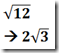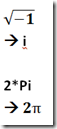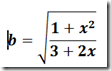Geeks With Blogs

The Mathematica http://www.wolfram.com/mathematica/ programming model consists of a kernel computation engine (or grid of such engines) and a front-end of notebook instances that communicate with the kernel throughout a session. The programming model of Mathematica is incredibly rich & powerful – besides numeric calculations, it supports symbols (eg Pi, I, E) and control flow logic.

obviously I could use this as a simple calculator:

5 * 10

--> 50

but this language is much more than that!

for example, I could use control flow logic & setup a simple infinite loop:

x=1;

While [x>0, x=x,x+1]

Different brackets have different purposes:

• square brackets for function arguments:  Cos[x]
• round brackets for grouping: (1+2)*3
• curly brackets for lists: {1,2,3,4}

The power of Mathematica (as opposed to say Matlab) is that it gives exact symbolic answers instead of a rounded numeric approximation (unless you request it):Mathematica lets you define scoped variables (symbols):

a=1;

b=2;

c=a+b

--> 5

these variables can contain symbolic values – you can think of these as partially computed functions:use Clear[x] or Remove[x] to zero or dereference a variable.

To compute a numerical approximation to n significant digits (default n=6), use N[x,n] or the //N prefix:

Pi //N

-->3.14159

N[Pi,50]

--> 3.1415926535897932384626433832795028841971693993751

The kernel uses % to reference the lastcalculation result, %% the

2nd last, %%% the 3rd last etc –> clearer statements:

Sqrt[Pi+Sqrt[Sqrt[Pi+Sqrt[Pi]]]

do:

Sqrt[Pi];

Sqrt[Pi+%];

Sqrt[Pi+%]

The help system supports wildcards, so I can search for functions like so:

?Inv*

Mathematica supports some very powerful programming constructs and a rich function library that allow you to do things that you would have to write allot of code for in a language like C++.

the Factor function – factorization:

Factor[x^3 – 6*x^2 +11x – 6]

--> (-3+x) (-2+x) (-1+x)

the Solve function – find the roots of an equation:

Solve[x^3 – 2x + 1 == 0]

the Expand function – express (1+x)^10 in polynomial form:

Expand[(1+x)^10]

--> 1+10x+45x^2+120x^3+210x^4+252x^5+210x^6+120x^7+45x^8+10x^9+x^10

the Prime function – what is the 1000th prime?

Prime

-->7919

Mathematica also has some powerful graphics capabilities:

the Plot function – plot the graph of y=Sin x in a single period:

Plot[Sin[x], {x,0,2*Pi}]

you can also plot 3D surfaces of functions using Plot3D function